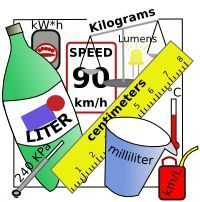Metric Measure (and Scientific Method)
3 years ago
bensonmike
Save
Edit
Host a game
Live GameLive
Homework
Solo Practice
Practice20 QuestionsShow answers
• Question 1
30 seconds
Q. What does the scientific method usually begin with?
finding data
analyzing data
a question
drawing a conclusion
• Question 2
30 seconds
Q. Why was the metric system created?
to pick a fight with another country
to standardize measurements
to create a competing measurement system
so that we have two systems to learn in school
• Question 3
30 seconds
Q. The International System (SI) is organized based on...
tens
hundreds
thousands
tenths
• Question 4
30 seconds
Q. When should you hypothesize when using the scientific method?
before you have a question
after you analyze data
after gathering data
after having a question or problem
• Question 5
30 seconds
Q. A good scientist should always communicate findings, regardless of the outcome of an experiment.
T or F?
True
False
Huh?
• Question 6
30 seconds
Q. The scientific instrument used for measuring weight...
pan balance
thermometer
spring scale
• Question 7
30 seconds
Q. The metric units used for mass...
ounces
grams
pounds
tons
• Question 8
30 seconds
Q. The type of measurement for measuring distance from one point to another...
altitude
circumference
length
weight
• Question 9
30 seconds
Q. The unit of measure typically used on a graduated cylinder...
mL
liter
ounce
kiloliter
• Question 10
30 seconds
Q. The base unit for volume...
milliliter
gram
milligram
liter
• Question 11
30 seconds
Q. The scientific instrument typically used to measure mass in our science class...
bathroom scale
balance
spring scale
triple-beam balance
• Question 12
30 seconds
Q. The amount matter in an object...
mass
weight
matter
atoms
• Question 13
30 seconds
Q. The instrument used to accurately measure liquid volume in a laboratory...
beaker
measuring spoon
measuring cup
• Question 14
30 seconds
Q. Kilo- means...
10
1000
0
100
• Question 15
30 seconds
Q. The prefix centi- means...
100
10
1000
.01
• Question 16
30 seconds
Q. The instrument used to measure time...
stopwatch
clock
watch
all of these choices are correct
• Question 17
30 seconds
Q. Units for the volume of a solid...
cubic centimeters
mL
cubic inches
grams
• Question 18
30 seconds
Q. Deci- means...
100
1000
1
0.10
• Question 19
30 seconds
Q. Weight measures...
the mass of an object
the pull of gravity on an object
how big you are
how old you are
• Question 20
30 seconds
Q. What unit of measure is used on a spring scale (for weight)?